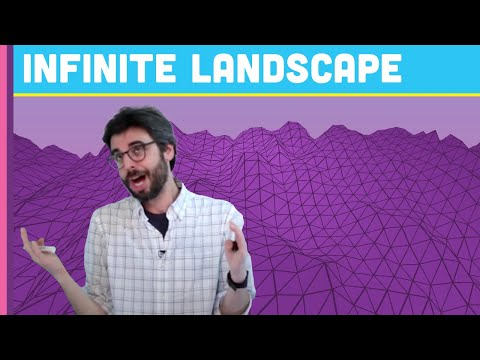#### Howdy, Stranger!

We are about to switch to a new forum software. Until then we have removed the registration on this forum.

# How to "project" or "map" 2D shapes or images on 3D terrain

edited June 2017

Hello,

a while ago I followed this Coding Challenge video by Daniel Shiffman to create a moving 3D terrain:

I now want to map a 2D shape onto the terrain. Unfortunately I am a complete noob to programming and have no idea how to do this so I would really appreciate some help.

Can someone please point me in the right direction? :)

Many thanks and best regards!

Tagged:

• Use a texture. Use the addition parameters to vertex().

``````// Daniel Shiffman
// http://codingtra.in
// http://patreon.com/codingtrain
// Code for:int cols, rows;
int scl = 20;
int w = 2000;
int h = 1600;

float flying = 0;

float[][] terrain;

PImage img;

void setup() {
size(600, 600, P3D);
cols = w / scl;
rows = h/ scl;
terrain = new float[cols][rows];
String http = "http://";
img = loadImage( http + "www.tfguy44.com/MyIcon1.PNG");
texture(img);
}

void draw() {

flying -= 0.1;

float yoff = flying;
for (int y = 0; y < rows; y++) {
float xoff = 0;
for (int x = 0; x < cols; x++) {
terrain[x][y] = map(noise(xoff, yoff), 0, 1, -100, 100);
xoff += 0.2;
}
yoff += 0.2;
}

background(0);
//stroke(255);
//noFill();

translate(width/2, height/2+50);
rotateX(PI/3);
scale(0.5);
translate(-w/2, -h/2);
for (int y = 0; y < rows-1; y++) {
beginShape(TRIANGLE_STRIP);
texture(img);
for (int x = 0; x < cols; x++) {
vertex(x*scl, y*scl, terrain[x][y], map(x,0,cols,0,img.width), map(y,0,rows,0,img.height) );
vertex(x*scl, (y+1)*scl, terrain[x][y+1], map(x,0,cols,0,img.width), map(y+1,0,rows,0,img.height));
//rect(x*scl, y*scl, scl, scl);
}
endShape();
}
}
``````
• Great! That's exactly what I was looking for! Thanks a lot! :)